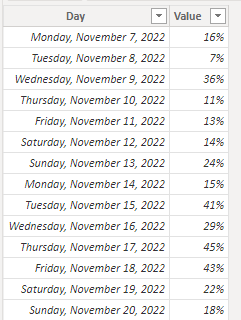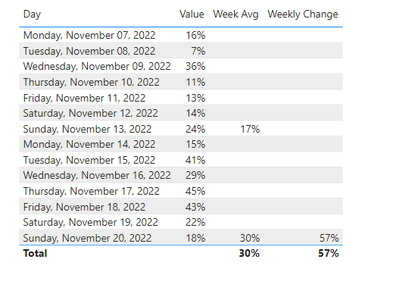cancel
Showing results for
Did you mean:Regular Visitor

## Grouped by week - determine change

Hi,

i have a problem where i stuck since several days, maybe you can help me.

I have a table with 2 columns, day and value. I'd like to get the value grouped bei a week Starting on Monday.

And i would like to determine the change of the weekly value compared to the previous week.

 Week Day Value Week Avg Weekly Change 1 Day 1 16% 1 Day 2 7% 1 Day 3 36% 1 Day 4 11% 1 Day 5 13% 1 Day 6 14% 1 Day 7 24% 17% / 2 Day 8 15% 2 Day 9 41% 2 Day 10 29% 2 Day 11 45% 2 Day 12 43% 2 Day 13 22% 2 Day 14 18% 30% 76%

Thank you in advance, i hope this is possible and there is a way to do this 🙂

best regards

Emil

1 ACCEPTED SOLUTIONCommunity Support

Hi @emildeak ,

Based on your description, I have created a simple sample:``````Week Avg =
var _a = WEEKDAY(MAX('Table'[Day]),2)
var _b = CALCULATE(AVERAGE('Table'[Value]),FILTER(ALL('Table'),WEEKNUM([Day],2)=WEEKNUM(MAX('Table'[Day]),2)))
return IF(_a=7,_b)

Weekly Change =
var _a = WEEKDAY(MAX('Table'[Day]),2)
var _b = CALCULATE(SUM('Table'[Value]),FILTER(ALL('Table'),WEEKNUM([Day],2)=WEEKNUM(MAX('Table'[Day]),2)))// the value of current week
var _c = CALCULATE(SUM('Table'[Value]),FILTER(ALL('Table'),WEEKNUM([Day],2)=WEEKNUM(MAX('Table'[Day]),2)-1))// the value of previous week
var _d = DIVIDE(_c,_b)
return IF(_a=7,_d)``````

Output:Since I'm not sure what your weekly change is based on, I calculated the percentage change in their total weekly value. You can change the aggregation in the measure to get the value you want, or clarify your calculation logic to me.

Best Regards,

Jianbo Li

If this post helps, then please consider Accept it as the solution to help the other members find it more quickly.

2 REPLIES 2Community Support

Hi @emildeak ,

Based on your description, I have created a simple sample:``````Week Avg =
var _a = WEEKDAY(MAX('Table'[Day]),2)
var _b = CALCULATE(AVERAGE('Table'[Value]),FILTER(ALL('Table'),WEEKNUM([Day],2)=WEEKNUM(MAX('Table'[Day]),2)))
return IF(_a=7,_b)

Weekly Change =
var _a = WEEKDAY(MAX('Table'[Day]),2)
var _b = CALCULATE(SUM('Table'[Value]),FILTER(ALL('Table'),WEEKNUM([Day],2)=WEEKNUM(MAX('Table'[Day]),2)))// the value of current week
var _c = CALCULATE(SUM('Table'[Value]),FILTER(ALL('Table'),WEEKNUM([Day],2)=WEEKNUM(MAX('Table'[Day]),2)-1))// the value of previous week
var _d = DIVIDE(_c,_b)
return IF(_a=7,_d)``````

Output:Since I'm not sure what your weekly change is based on, I calculated the percentage change in their total weekly value. You can change the aggregation in the measure to get the value you want, or clarify your calculation logic to me.

Best Regards,

Jianbo Li

If this post helps, then please consider Accept it as the solution to help the other members find it more quickly.Super User

@emildeak , one of the ways is to have week rank on the year week column, in separate week/date table

new column

Week Rank RANKX(all('Date'),'Date'[Year Week],,ASC,Dense) //YYYYWW format

This Week = CALCULATE(Average('Table'[Value]), FILTER(ALL('Date'),'Date'[Week Rank]=max('Date'[Week Rank])))
Last Week = CALCULATE(Average('Table'[Value]), FILTER(ALL('Date'),'Date'[Week Rank]=max('Date'[Week Rank])-1))